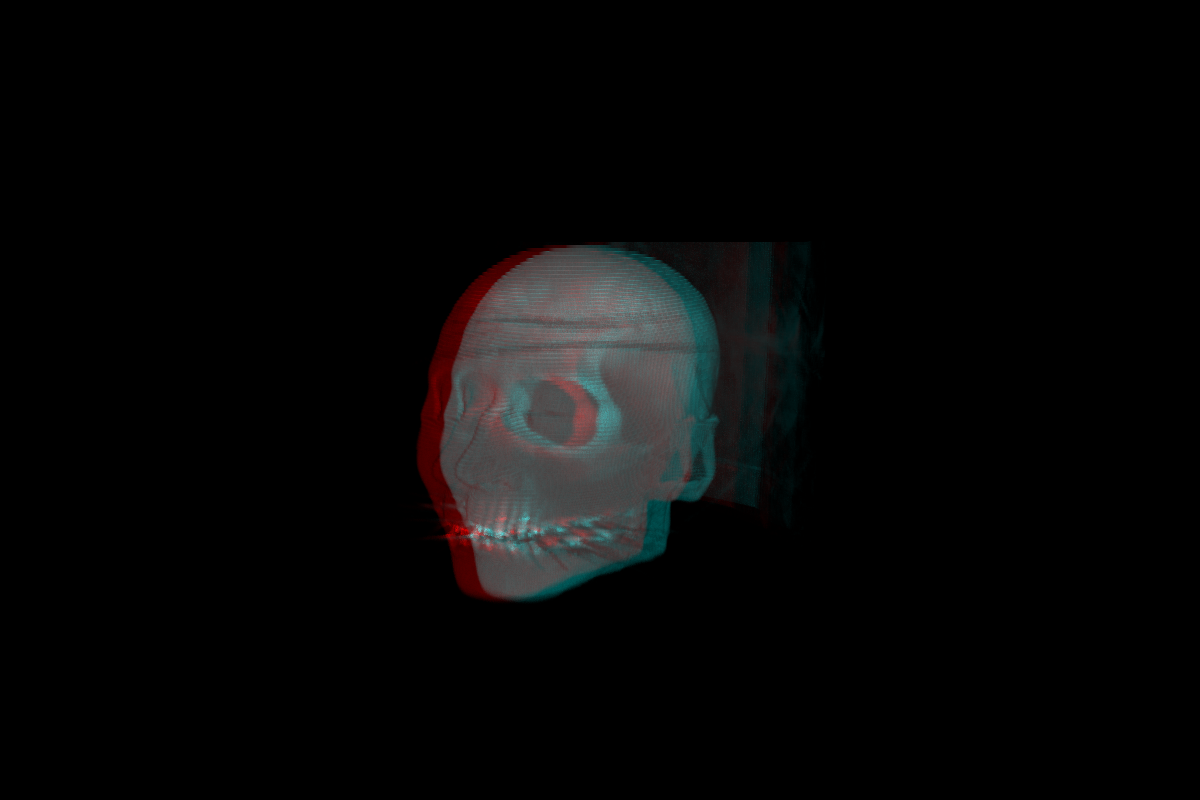• ### Ofelia Volumetrics Example

Here is the patch and an explanation how to add addons to Ofelia:
https://github.com/Jonathhhan/volumetrics_example_ofelia
Maybe this is a good foundation for making a 3d game of life that runs on a shader...
A funny thing (but good to know), that took a lot of time, was that it is not allowed to use function names in ofxOfeliaPdBindings.h that include "upd" - and i was using updateVolumeData() from the addon. I had to change it to loadVolumeData (or something else without "upd").

• Posts 5 | Views 1266
• Second example, basically the same patch, but with ofxCameraAnaglyph for a 3d view...
https://github.com/Jonathhhan/volumetrics_anaglyph_example_ofelia
Here is a screenshot:• I tried to create the shader for a 3d game of life (adapted from my working 2d game of life shader https://github.com/Jonathhhan/PDGameofLife-shaderVersion-):

``````#version 120

uniform sampler3D Tex0;
uniform vec3 resolution;
uniform float lCell_0;
uniform float lCell_1;
uniform float lCell_2;
uniform float lCell_3;
uniform float lCell_4;
uniform float lCell_5;
uniform float lCell_6;
uniform float lCell_7;
uniform float lCell_8;
uniform float lCell_9;
uniform float lCell_10;
uniform float lCell_11;
uniform float lCell_12;
uniform float lCell_13;
uniform float lCell_14;
uniform float lCell_15;
uniform float lCell_16;
uniform float lCell_17;
uniform float lCell_18;
uniform float lCell_19;
uniform float lCell_20;
uniform float lCell_21;
uniform float lCell_22;
uniform float lCell_23;
uniform float lCell_24;
uniform float lCell_25;
uniform float lCell_26;
uniform float dCell_0;
uniform float dCell_1;
uniform float dCell_2;
uniform float dCell_3;
uniform float dCell_4;
uniform float dCell_5;
uniform float dCell_6;
uniform float dCell_7;
uniform float dCell_8;
uniform float dCell_9;
uniform float dCell_10;
uniform float dCell_11;
uniform float dCell_12;
uniform float dCell_13;
uniform float dCell_14;
uniform float dCell_15;
uniform float dCell_16;
uniform float dCell_17;
uniform float dCell_18;
uniform float dCell_19;
uniform float dCell_20;
uniform float dCell_21;
uniform float dCell_22;
uniform float dCell_23;
uniform float dCell_24;
uniform float dCell_25;
uniform float dCell_26;

vec2 rule = vec2(
vec2(lCell_0, dCell_0),
vec2(lCell_1, dCell_1),
vec2(lCell_2, dCell_2),
vec2(lCell_3, dCell_3),
vec2(lCell_4, dCell_4),
vec2(lCell_5, dCell_5),
vec2(lCell_6, dCell_6),
vec2(lCell_7, dCell_7),
vec2(lCell_8, dCell_8),
vec2(lCell_9, dCell_9),
vec2(lCell_10, dCell_10),
vec2(lCell_11, dCell_11),
vec2(lCell_12, dCell_12),
vec2(lCell_13, dCell_13),
vec2(lCell_14, dCell_14),
vec2(lCell_15, dCell_15),
vec2(lCell_16, dCell_16),
vec2(lCell_17, dCell_17),
vec2(lCell_18, dCell_18),
vec2(lCell_19, dCell_19),
vec2(lCell_20, dCell_20),
vec2(lCell_21, dCell_21),
vec2(lCell_22, dCell_22),
vec2(lCell_23, dCell_23),
vec2(lCell_24, dCell_24),
vec2(lCell_25, dCell_25),
vec2(lCell_26, dCell_26)
);

int get(int x, int y, int z) {
return int(texture3D(Tex0, (gl_FragCoord.xyz + vec3(x, y, z)) / resolution).r);
}

void main() {
int sum =
get(-1, -1, -1) +
get(-1,  0, -1) +
get(-1,  1, -1) +
get( 0, -1, -1) +
get( 0,  1, -1) +
get( 1, -1, -1) +
get( 1,  0, -1) +
get( 1,  1, -1) +
get(-1, -1, 0) +
get(-1,  0, 0) +
get(-1,  1, 0) +
get( 0, -1, 0) +
get( 0,  1, 0) +
get( 1, -1, 0) +
get( 1,  0, 0) +
get( 1,  1, 0) +
get(-1, -1, 1) +
get(-1,  0, 1) +
get(-1,  1, 1) +
get( 0, -1, 1) +
get( 0,  1, 1) +
get( 1, -1, 1) +
get( 1,  0, 1) +
get( 1,  1, 1);
vec2 r = rule[sum];
if (get(0, 0, 0) == 1) {
gl_FragColor = vec4(r.x, r.x, r.x, r.x);
}
else  {
gl_FragColor = vec4(r.y, r.y, r.y, r.y);
}
``````

But it does not work.Perhaps I did something wrong with the shader, but I think it does not work because ofFbo() can not store 3d textures.

• Very cool to see some of Ofelia's extensibility -- and nice to see more shader examples (even if 3d seems not fully supported yet).

hjh

• @ddw_music i think the 3d game of life shader works now. not that it is not supported, i just did not understand it.The only thing is, that ofFbo and ofTexture do not support 3d textures, but they need to be created with openGL (as far as I know, and what I did with help from ofxVolumetrics).
I only did not find a way to paint into the 3d texture yet, right know I need a .png image for every z dimension.
Here is a short example:

https://github.com/Jonathhhan/PureDataGameOfLife3d

Posts 5 | Views 1266
Internal error.

Oops! Looks like something went wrong!